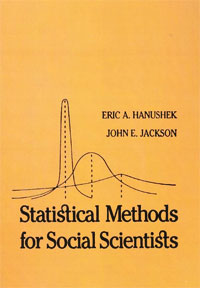Skip to content Skip to navigation

# Statistical Methods for Social Scientists

Author/s:
Eric A. Hanushek, John E. Jackson
Published Date:
1977
Publication:
New York: Academic Press
Pages:
374 pagesThe aspects of this text which we believe are novel, at least in degree, include: an effort to motivate different sections with practical examples and an empirical orientation; an effort to intersperse several easily motivated examples throughout the book and to maintain some continuity in these examples; and the extensive use of Monte Carlo simulations to demonstrate particular aspects of the problems and estimators being considered. In terms of material being presented, the unique aspects include the first chapter which attempts to address the use of empirical methods in the social sciences, the seventh chapter which considers models with discrete dependent variables and unobserved variables. Clearly these last two topics in particular are quite advanced--more advanced than material that is currently available on the subject. These last two topics are also currently experiencing rapid development and are not adequately described in most other texts.

 CONTENTS 1 Empirical Analyses in the Social Sciences Introduction Social Science Theory and Statistical Models Fitting Models to Data The Development of Stochastic Model The Analysis of Nonexperimental Data and the Selection of a Statistical Procedure Simple Methods Review Questions 2 Estimation with Simple Linear Models Introduction     The Basic Model                                                                                      Least Squares Estimators                Two Examples             Conclusion               Appendix: Properties of Summations             Appendix: Calculus and the Minimization of Functions                Review Questions 3 Least Squares Estimators: Statistical Properties and Hypothesis Testing Introduction Properties of Least Squares Estimators Distribution of b-A Monte Carlo Experiment             Statistical Inference                            Hypothesis Tests for Schooling/Earnings Model              Conclusion                                      Appendix: Estimation of Schooling/Earnings Model Using SPSS Computer Program Review Questions 4 Ordinary Least Squares in Practice Introduction             Interpretation of Regression Coefficients              Model Specification                                    Model Specification and Multicollinearity in Practice            Functional Forms                                       Dummy Explanatory Variables                            Review Questions 5 Multivariate Estimation in Matrix Form Introduction                                     The Least Squares Estimators                                    Least Squares in Matrix Notation                                Properties of Least Squares                                     Distributional Aspects of the Error Term                                  Statistical Inference               Multivariate Education Example                                  Multicollinearity                                Conclusion                                       Appendix: Proof of Best                          Appendix: Proof of Unbiasedness of the Estimator of Variance Review Questions 6 Generalized Least Squares Introduction             Heteroskedasticity and Autocorrelation            Formal Statement of the Problem                   Generalized Least Squares                         Generalized Least Squares and Examples of Heteroskedasticity and Autocorrelation              Generalized Least Squares and Weighted Regression                Monte Carlo Simulation of Generalized Least Squares Generalized Least Squares in Practice                       Visual Diagnostics                                          Dynamic Models                                              Conclusion                                                  Appendix: Derivation of Generalized Least Squares Estimator                                      Appendix: Unbiased Estimator of Variance Review Questions 7 Models with Discrete Dependent Variables Introduction The Problem of Estimating Models with Discrete Dependent Variables                               Alternative Models-Dichotomous Dependent Variables               Logit Analysis grouped Data                                 Logit Analysis-Microdata                                    Probit Analysis                                             An Example                                                  Monte Carlo Simulation of Dichotomous Dependent Variables             Polytomous Variables/Joint Distributions                    Conclusions 8 Introduction to Multiequation Models Introduction Two Examples of Structural Systems Path Analysis The General Multiequation Model Estimating Hierarchical Models Hierarchical, Nonrecursive Systems Underidentification in Hierarchical Models Nonrecursive Hierarchical Models: Two Examples Conclusion Appendix: Instrumental Variables Estimator Review Questions 9 Structural Equations: Simultaneous Models Introduction             Identification in Simultaneous Systems: An Example                 Identification in Simultaneous Models             Estimating Identified Models                      Simultaneous Equations: The Voting and Aspiration Examples            Identification through Assumptions about Error Terms             Alternative Estimators             Summary and Conclusions            Appendix: Variances and Covariances for Peer Influence Data                       Review Questions 10 Estimating Models with Erroneous and Unobserved Variables Introduction Erroneous Explanatory Variables Unobserved Variables Factor Analysis Linear Structural Models and the General Analysis of Covariances Conclusion Appendix I Statistical Review Probability Theoretical Distributions Properties of Estimators Hypothesis Testing Maximum Likelihood (ML) Estimation Appendix II Matrix Algebra Basic Properties Basic Operations Matrix Multiplication Other Operations Systems of Linear Equations Inverses Existence of an Inverse-Rank Review Questions for Appendix 11 Appendix III Statistical Tables References References
Topics: